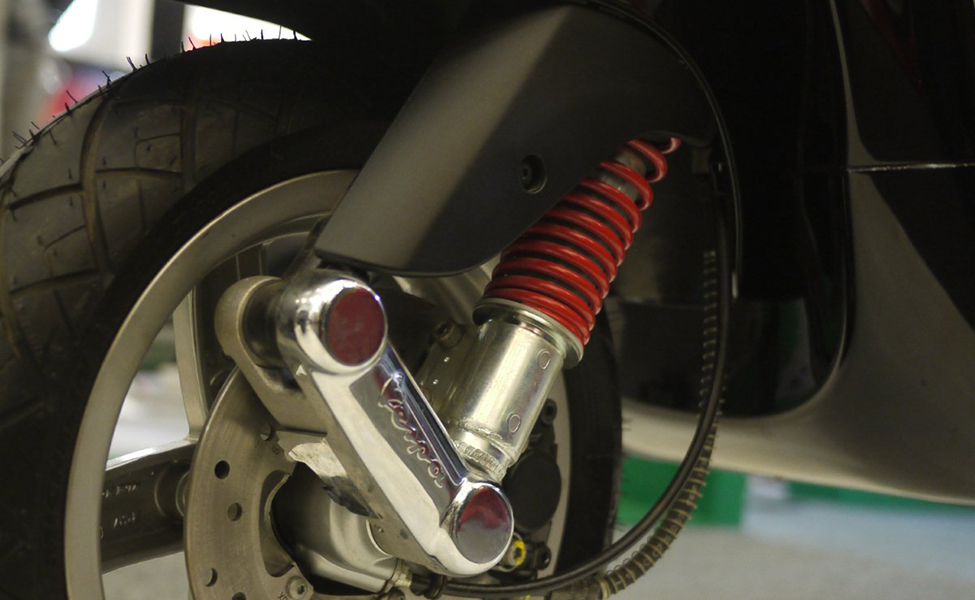Second-Order Differential Equations

# 46 Introduction

A motorcycle suspension system is an example of a damped spring-mass system. The spring absorbs bumps and keeps the tire in contact with the road. The shock absorber damps the motion so the motorcycle does not continue to bounce after going over each bump. (credit: nSeika, Flickr)We have already studied the basics of differential equations, including separable first-order equations. In this chapter, we go a little further and look at second-order equations, which are equations containing second derivatives of the dependent variable. The solution methods we examine are different from those discussed earlier, and the solutions tend to involve trigonometric functions as well as exponential functions. Here we concentrate primarily on second-order equations with constant coefficients.

Such equations have many practical applications. The operation of certain electrical circuits, known as resistor–inductor–capacitor (RLC) circuits, can be described by second-order differential equations with constant coefficients. These circuits are found in all kinds of modern electronic devices—from computers to smartphones to televisions. Such circuits can be used to select a range of frequencies from the entire radio wave spectrum, and are they commonly used for tuning AM/FM radios. We look at these circuits more closely in Applications.

Spring-mass systems, such as motorcycle shock absorbers, are a second common application of second-order differential equations. For motocross riders, the suspension systems on their motorcycles are very important. The off-road courses on which they ride often include jumps, and losing control of the motorcycle when landing could cost them the race. The movement of the shock absorber depends on the amount of damping in the system. In this chapter, we model forced and unforced spring-mass systems with varying amounts of damping.